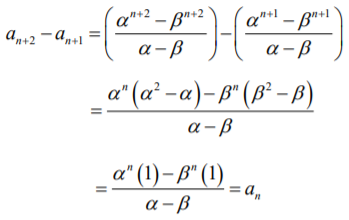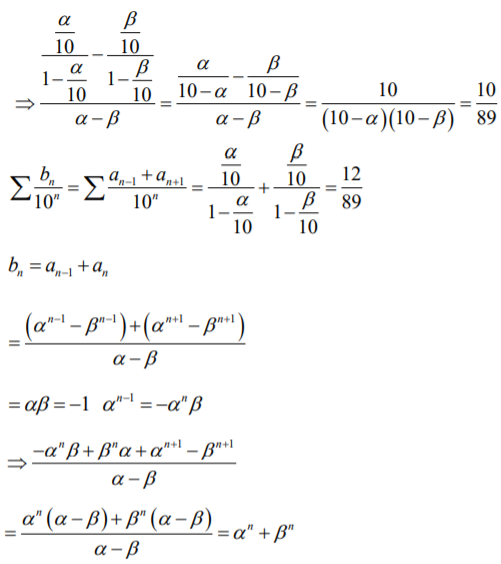Checkout JEE MAINS 2022 Question Paper Analysis : Checkout JEE MAINS 2022 Question Paper Analysis :

# Let α and β be the roots of x2 – x – 1 = 0....

Let α and β be the roots of x2 – x – 1 = 0, with α > β. For all positive integers n, define

$$\begin{array}{l}a_n = \frac{\alpha^n – \beta^n}{\alpha – \beta}, n \geq 1\end{array}$$

b1 = 1 and bn = an-1 + an+1, n ≥ 2.

Then which of the following options is/are correct?

a)

$$\begin{array}{l}a_1 + a_2 + a_3 +….+a_n = a_{n+2} – 1\end{array}$$
for all n ≥ 1

b)

$$\begin{array}{l}\sum_{n=1}^\infty \frac{a_n}{10^n} = \frac{10}{89}\end{array}$$

c)

$$\begin{array}{l}\sum_{n=1}^\infty \frac{b_n}{10^n} = \frac{8}{89}\end{array}$$

d)

$$\begin{array}{l}b_n = \alpha^n + \beta^n\end{array}$$
for all n ≥ 1.

Solution:

x2 – x – 1 = 0

α = [1+ √5]/2 and β = [1- √5]/2

$$\begin{array}{l}a_n = \frac{\alpha^n – \beta^n}{\alpha – \beta}\end{array}$$

As, b1 = 1 and bn = an-1 + an+1, n ≥ 2a1 + a2 + …+ an =

$$\begin{array}{l}\sum_{n=1}^\infty \frac{a_n}{10^n}\end{array}$$

=> an + an+1 = an+2

=>

$$\begin{array}{l}\frac{\sum (\frac{\alpha}{10})^n – \sum(\frac{\beta}{10})^n}{\alpha – \beta}\end{array}$$

$$\begin{array}{l}\sum_{r=1}^n a_r= a_{n+2} – a_2\end{array}$$

= an+2 – [α2 – β2]/[α -β]

= an+2 – [α + β]

= an+2 – 1(0)(2)